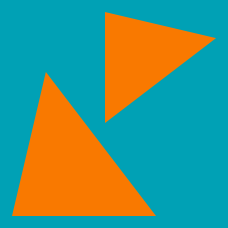Geometry

# Congruent and Similar Triangles: Level 1 Challenges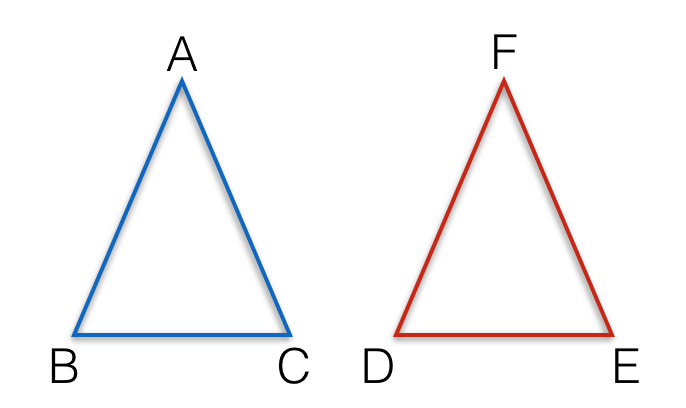In triangles $ABC$ and $DEF$, if we know that $AB = EF, BC = DE$ and $\angle ABC = \angle DEF$, are the triangles congruent?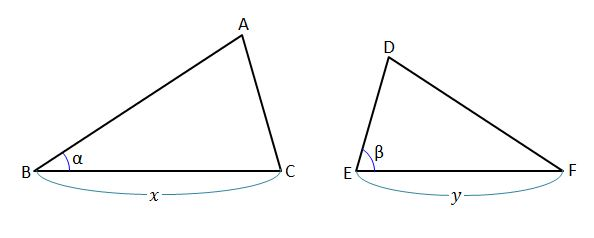In the above diagram, $x=21,$ $y=14,$ $\alpha={35}^\circ,$ and $\beta={75}^\circ.$ Which condition would mean that the two triangles are similar?

Note: The above diagram is not drawn to scale.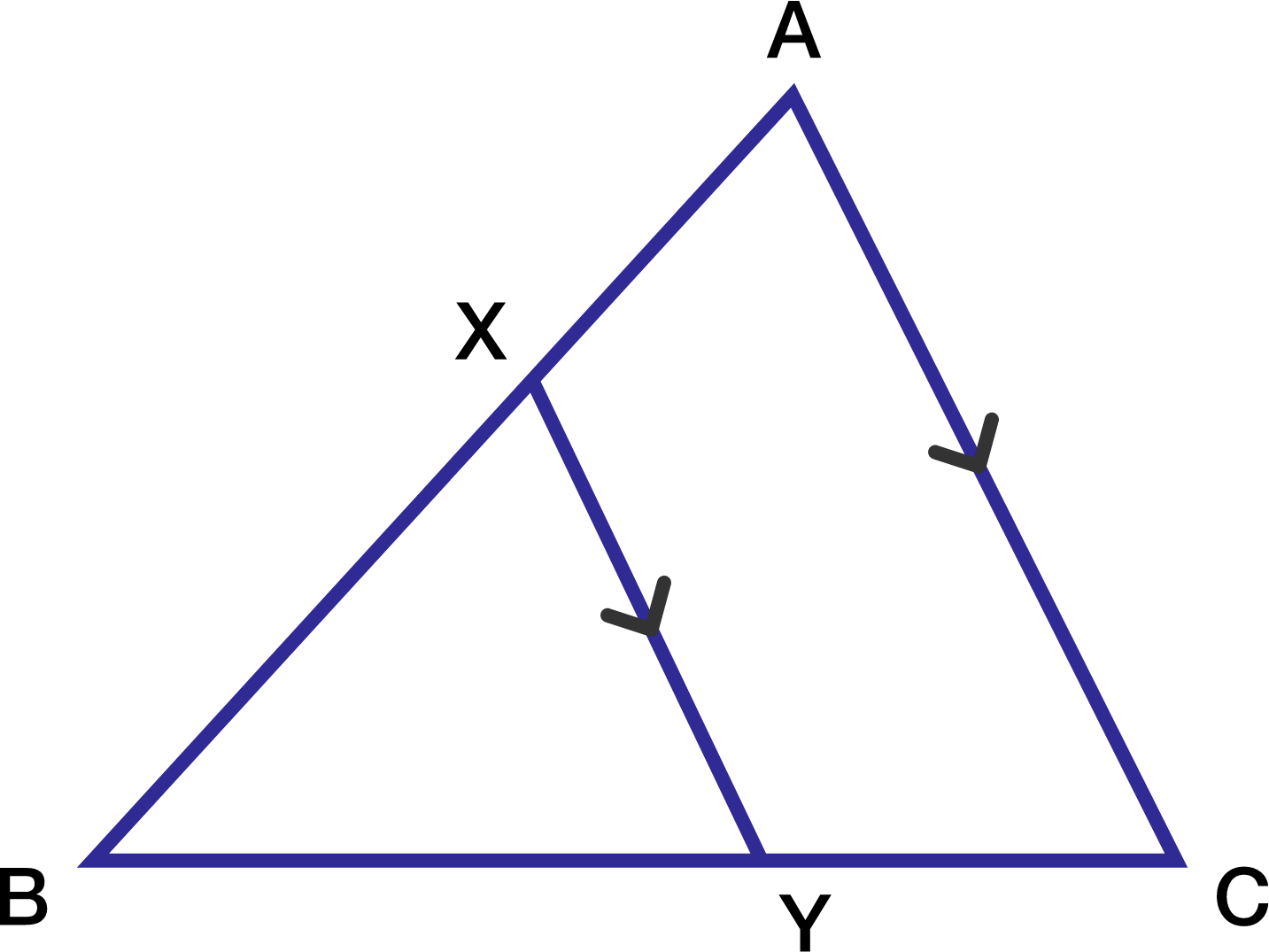In the adjoining figure, $XY$ is parallel to $AC$. If $XY$ divides the triangle into two halves with equal area, compute $\dfrac{AX}{AB}$.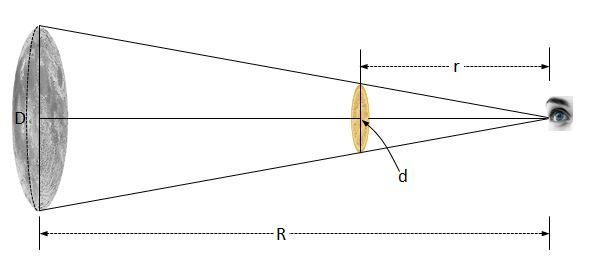Did you know you can approximate the diameter of the moon with a coin $($of diameter $d)$ placed a distance $r$ in front of your eye?

If the distance between the moon and your eye is $R,$ what is the diameter of the moon?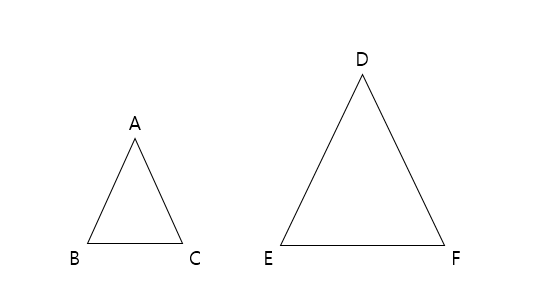Triangle $\triangle ABC$ is similar to $\triangle DEF$, and the ratio of their areas is $9:25.$ If the length of $\overline{DE}$ is $60,$ what is the length of $\overline{AB}$?

×